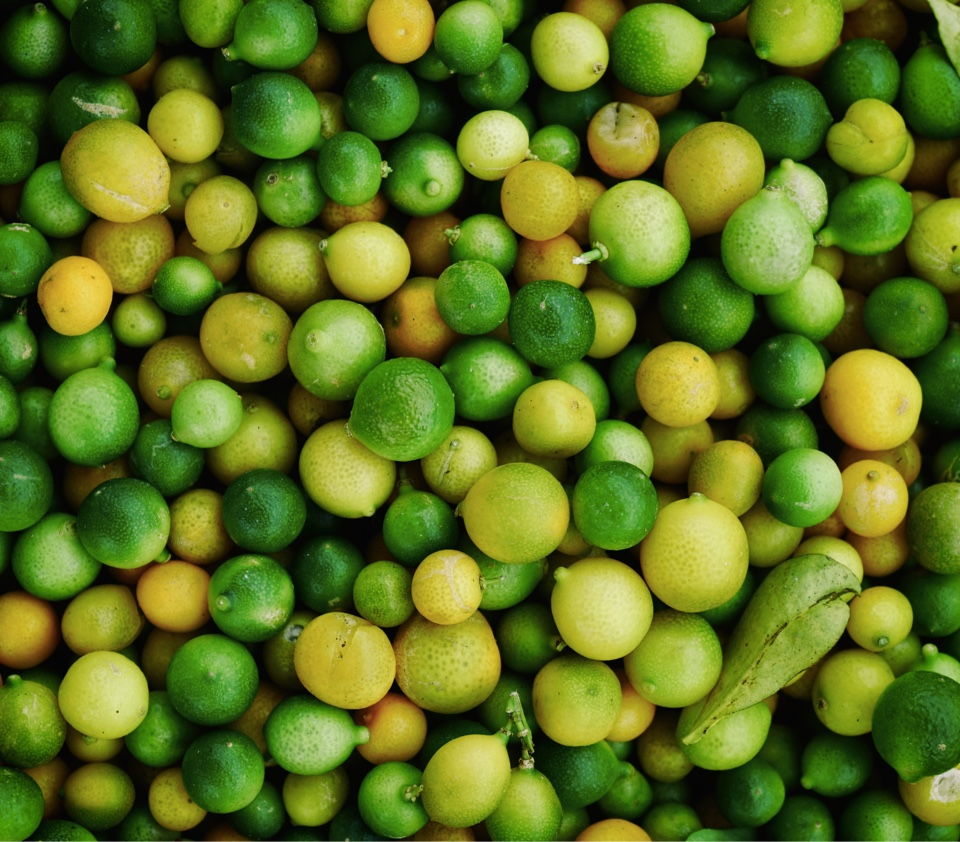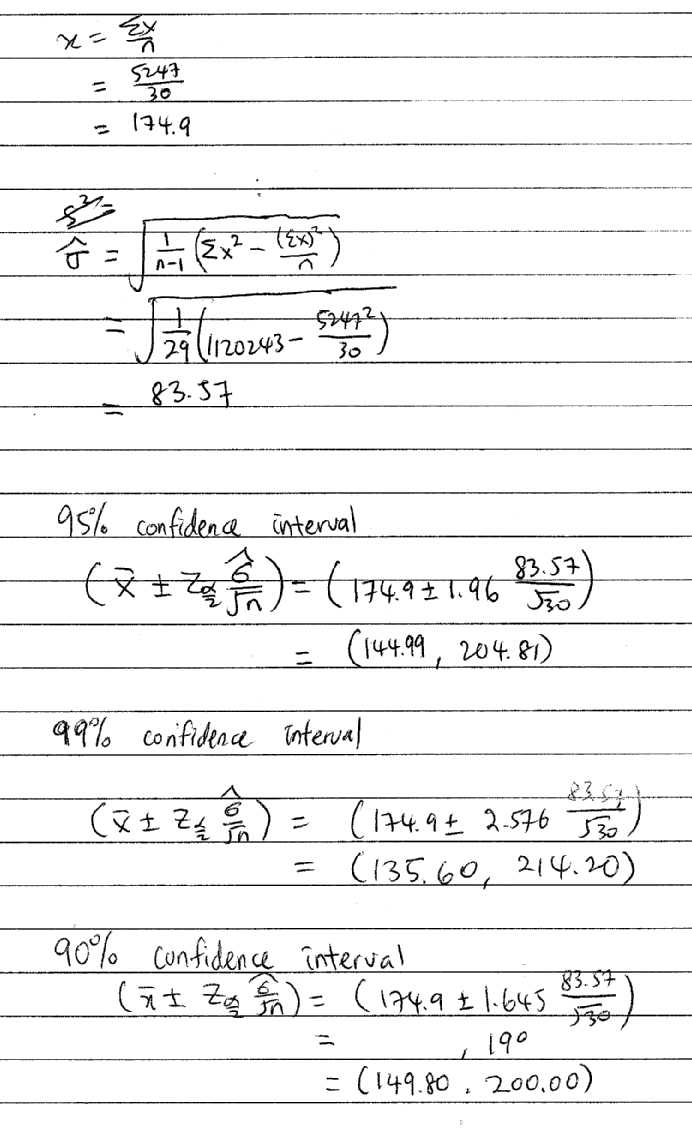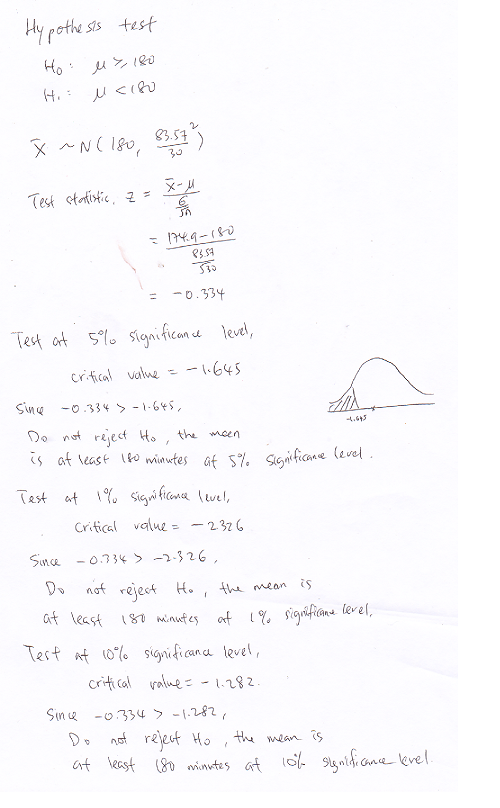Select PageSTPM 2017 Term 3 MT Coursework Explained.

“Only sample solution for mathematical part will be posted. Please ask your school teacher for introduction, methodology, and conclusion.”##### —00

It  is  reported  that  Malaysian  youths  spend  many  hours  daily  to  access  the  social  media  such  as Facebook and WhatsApp.  This phenomenon leads to deterioration of social ties and values.

In this assignment, you are required to conduct a study on the time spent daily by the students in your school to access the social media.##### —01

(a)
Discuss the various sampling techniques that can be used to collect data.

(b)
Choose a suitable sampling technique to collect data on time spent daily (in minutes) for a random sample of n students.

##### —02

Construct three confidence intervals for the mean time spent daily by students in your school to access the social media. Interpret your results.##### —03

Determine  whether  the  average  time  spent  daily  by  the  students  in  your  school  to  access  the
social media is at least three hours.

##### —04

State any assumptions made in Task 2 and Task 3. Discuss the validity of your results.Sample Solution

##### —01(a)

Reference: https://onlinecourses.science.psu.edu/stat100/node/18

Please find other resources yourself.

Sampling Methods can be classified into one of two categories:

Probability Sampling: Sample has a known probability of being selected
Non-probability Sampling: Sample does not have known probability of being selected as in convenience or voluntary response surveys

Probability Sampling

In probability sampling it is possible to both determine which sampling units belong to which sample and the probability that each sample will be selected. The following sampling methods are examples of probability sampling:

1. Simple Random Sampling (SRS)
2. Stratified Sampling
3. Cluster Sampling
4. Systematic Sampling
5. Multistage Sampling (in which some of the methods above are combined in stages)

Of the five methods listed above, students have the most trouble distinguishing between stratified sampling and cluster sampling.

Stratified Sampling is possible when it makes sense to partition the population into groups based on a factor that may influence the variable that is being measured. These groups are then called strata. An individual group is called a stratum. With stratified sampling one should:

• partition the population into groups (strata)
• obtain a simple random sample from each group (stratum)
• collect data on each sampling unit that was randomly sampled from each group (stratum)

Stratified sampling works best when a heterogeneous population is split into fairly homogeneous groups. Under these conditions, stratification generally produces more precise estimates of the population percents than estimates that would be found from a simple random sample.

Cluster Sampling is very different from Stratified Sampling. With cluster sampling one should

• divide the population into groups (clusters).
• obtain a simple random sample of so many clusters from all possible clusters.
• obtain data on every sampling unit in each of the randomly selected clusters.

It is important to note that, unlike with the strata in stratified sampling, the clusters should be microcosms, rather than subsections, of the population.   Each cluster should be heterogeneous. Additionally, the statistical analysis used with cluster sampling is not only different, but also more complicated than that used with stratified sampling.

Non-probability Sampling

The following sampling methods that are listed in your text are types of non-probability sampling that should be avoided:

1. volunteer samples
2. haphazard (convenience) samples

Get more references from Internet.

##### —01 (b)

Define the survey form you used properly.

Random sampling is the purest form of probability sampling. Each member of the population has an equal and known chance of being selected. When there are very large populations, it is often difficult or impossible to identify every member of the population, so the pool of available subjects becomes biased.

A random sample of  30 students is collected from the school (describe in details). The data on time spent daily by students in school to access the social media is recorded.

You can prepare a survey form to get the data of the 30 students.

Below are parts of the data collected. You can also include the info (name, interest, and others) of the respondent.

222
289
204
133
244
194
257
59
192
294
221
278
38
177
9
190
279
11
160
176
202
178
105
221
177
13
182
249
89
204

##### —02

You can construct three confidence intervals with different confidence levels.There is a probability of 0.95 that the mean time spent daily by students in school to access the social media lies between 144.99 minutes and 204.81 minutes.

##### —03

Using hypothesis test with 5% (1%, 10%) significance level.##### —04

Assumption made

1. The data are sampled randomly because it avoids bias.

2. The sample values are independent of each other.

3. The data are collected from a random sample from large population (all students from KL).

4. The sample size is sufficiently large. Although the Central Limit Theorem tells us that we can use a Normal model to think about the behavior of sample means when the sample size is large enough, it does not tell us how large that should be. If the population is very skewed, you will need a pretty large sample size to use the CLT, however if the population is unimodal and symmetric, even small samples are ok. So think about your sample size in terms of what you know about the population and decide whether the sample is large enough. In general a sample size of 30 is considered sufficient.

5. The sampling distribution is normally distributed. The population is normally distributed.

6. Standard distribution is assumed as above formula.## Subscribe To KK LEE Newsletter

Join KKL EE mailing list to receive the latest news and updates from the team. Get notified when new articles (including pbs sample) are posted.